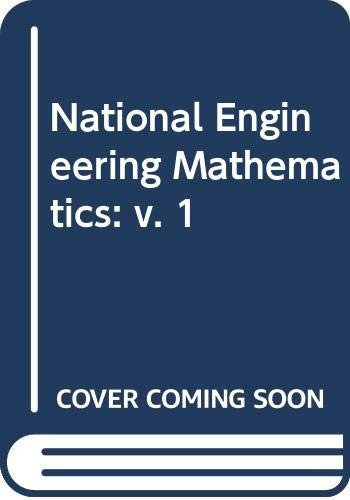# National Engineering Mathematics by J.C. Yates

Out of Stock
\$18.49
inc. GST
This text is designed for all BTEC National Engineering and Science students. The first volume covers the Level II maths syllabus including a thorough introduction to calculus. The book is assignment driven, with each topic built around a significant practical application.
Only 0 left

## National Engineering Mathematics Summary

### National Engineering Mathematics: v. 1 by J.C. Yates

This text is designed for all BTEC National Engineering and Science students. The first volume covers the Level II maths syllabus including a thorough introduction to calculus. The book is assignment driven, with each topic built around a significant practical application. The learner works step-by-step through 1229 worked examples and exercises, so the book can be used for self study and workshop applications.

### Why buy from World of BooksOur excellent value books literally don't cost the earthFree delivery in AustraliaEvery used book bought is one saved from landfill

Acknowledgements - Author's Note - The Electronic Calculator - Linear Equations and Straight Line Graphs - Transposition of Formulae - Circular Measure - Trigonometry: I - Trigonometry: II - Quadratic Equations - Cubic Equations - Simultaneous Equations - Indices and Logarithms - Graphs of Logarithms and Exponentials - Further Graphs - Vectors - Detrimants and Matrices - Series - Surface Areas and Volumes - Statistics - Calculus: I; Differentiation - Calculus: II; Integration - Answers to Exercises - Index

GOR004287129
National Engineering Mathematics: v. 1 by J.C. Yates
J.C. Yates